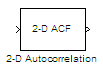Documentation

# 2-D Autocorrelation

Compute 2-D autocorrelation of input matrix

## Library

Statistics

`visionstatistics`## Description

The 2-D Autocorrelation block computes the two-dimensional autocorrelation of the input matrix. Assume that input matrix A has dimensions (Ma, Na). The equation for the two-dimensional discrete autocorrelation is

`$C\left(i,j\right)=\sum _{m=0}^{\left(Ma-1\right)}\sum _{n=0}^{\left(Na-1\right)}A\left(m,n\right)\cdot conj\left(A\left(m+i,n+j\right)\right)$`

where $0\le i<2Ma-1$ and $0\le j<2Na-1$.

The output of this block has dimensions $\left(2Ma-1,2Na-1\right)$.

PortInput/OutputSupported Data TypesComplex Values Supported

Input

Vector or matrix of intensity values or a scalar, vector, or matrix that represents one plane of the RGB video stream

• Double-precision floating point

• Single-precision floating point

• Fixed point

• 8-, 16-, 32-bit signed integer

• 8-, 16-, 32-bit unsigned integer

Yes

Output

Autocorrelation of the input matrix

Same as Input port

Yes

If the data type of the input is floating point, the output of the block has the same data type.

### Fixed-Point Data Types

The following diagram shows the data types used in the 2-D Autocorrelation block for fixed-point signals.You can set the product output, accumulator, and output data types in the block mask as discussed in Parameters.

The output of the multiplier is in the product output data type if at least one of the inputs to the multiplier is real. If both of the inputs to the multiplier are complex, the result of the multiplication is in the accumulator data type. For details on the complex multiplication performed, refer to Multiplication Data Types.

## Parameters

Rounding mode

Select the Rounding Modes for fixed-point operations.

Saturate on integer overflow

Select the overflow mode for fixed-point operations. See Precision and Range.

Product output

Specify the product output data type. See Fixed-Point Data Types and Multiplication Data Types for illustrations depicting the use of the product output data type in this block:

• When you select `Same as input`, these characteristics match those of the input to the block.

• When you select `Binary point scaling`, you can enter the word length and the fraction length of the product output, in bits.

• When you select `Slope and bias scaling`, you can enter the word length, in bits, and the slope of the product output. The bias of all signals in the Computer Vision Toolbox™ software is 0.

Accumulator

Use this parameter to specify how to designate the accumulator word and fraction lengths. Refer to Fixed-Point Data Types and Multiplication Data Types for illustrations depicting the use of the accumulator data type in this block. The accumulator data type is only used when both inputs to the multiplier are complex.

• When you select `Same as product output`, these characteristics match those of the product output.

• When you select `Same as input`, these characteristics match those of the input to the block.

• When you select `Binary point scaling`, you can enter the word length and the fraction length of the accumulator, in bits.

• When you select `Slope and bias scaling`, you can enter the word length, in bits, and the slope of the accumulator. The bias of all signals in the Computer Vision Toolbox software is `0`.

Output

Choose how to specify the output word length and fraction length.

• When you select `Same as input`, these characteristics match those of the input to the block.

• When you select `Binary point scaling`, you can enter the word length and the fraction length of the output, in bits.

• When you select `Slope and bias scaling`, you can enter the word length, in bits, and the slope of the output. The bias of all signals in the Computer Vision Toolbox software is `0`.

Lock data type settings against change by the fixed-point tools

Select this parameter to prevent the fixed-point tools from overriding the data types you specify on the block mask. For more information, see `fxptdlg`, a reference page on the Fixed-Point Tool in the Simulink® documentation.

## See Also

 2-D Correlation Computer Vision Toolbox 2-D Histogram Computer Vision Toolbox 2-D Mean Computer Vision Toolbox 2-D Median Computer Vision Toolbox 2-D Standard Deviation Computer Vision Toolbox 2-D Variance Computer Vision Toolbox 2-D Maximum Computer Vision Toolbox 2-D Minimum Computer Vision Toolbox

Download ebook